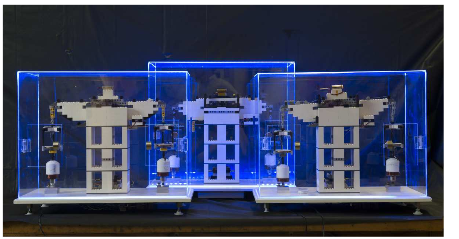# Boffins weigh in to perfect kilogram quest with LEGO kit

## Bricked-up watt balance is 99 per cent accurate

How many boffins does it take to make a LEGO machine to measure a fundamental physical unit? Nine, apparently – but the outcome, a LEGO watt balance, can measure a kilogram with about one per cent inaccuracy.

The serious point to the National Institute of Standards and Technology-led effort (NIST) is that with the Le Système International d'Unités (SI) definition of the kilogram under review, the researchers have produced a handy way to explain how the kilogram can be defined with reference only to the quantum value known as the Planck constant "h".

Their watt balance allows the measurement of weight without needing reference back to the current SI standard, the International Prototype Kilogram (IPK). As they explain in their Arxiv paper (soon to land in the American Journal of Physics), the LEGO watt balance is calibrated “solely in terms of electrical, optical, and frequency measurements”.Three versions of the NIST-designed LEGO watt balance

The basis of the watt balance is that the electromagnetic forces acting on an object can be precisely predicted, allowing the unknown mass to be measured not by comparison to something tested against the IPK, but on electromagnetic theory alone.

As the researchers from NIST, MIT and the University of Maryland explain, by measuring first the voltage induced by the movement of a coil through a magnetic field (velocity mode) and then the force needed to hold a mass still, a simple enough calculation provides a mass measurement traceable to the Planck constant.

In velocity mode, a coil of length L moves at a vertical speed v through a magnetic field (flux density B). The induced voltage V equals BLv. In force mode, the (known) gravitational force on a suspended mass is in opposition to a current I in the coil, in the equation F = BLI = mg (mg being the product of mass and gravity). Since BL is common to the two equations, it cancels, and that provides the calculation of the unknown mass: m = VI/gv (that is, the measured induced voltage multiplied by the current, divided by the product of gravitational acceleration and velocity).

The Josephson effect and the Hall effect then provide the means to trace the measured mass to the Planck constant, the paper explains.

Sadly, the boffins couldn't manage to come up with a purely LEGO construction, since the toy's inventory doesn't include neodymium ring magnets, 1-inch PVC pipe or AWG-35 wire, but that's a minor quibble compared to letting schools measure the Planck constant (see page three of the paper) without too much outlay.

It's also pretty neat to be able to show off the relationship between a quantum value and the macroscopic world, and “it is also interesting to consider how an apparatus assembled from plastic bricks can measure h in a classroom or living room setting with uncertainty of only 1 per cent”.

The paper includes part-list and CAD diagrams, and if you were starting from scratch the full kit comes in at around US\$600. ®

Send us news